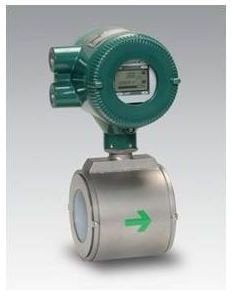# Magnetic Flow Meter: Measurement & Calculations of Flow Rate

Page content

## Introduction

Pipe flow rate - how to measure it? There are indeed many flow meter choices to use for pipe flow measurement. This series on measurement of pipe flow rate gives information about several types of flow meters, and this article gives details about one of them, the magnetic flow meter, including general principles of its operation and its advantages and disadvantages in comparison with other types of pipe flow meter. The magnetic flow meter is the third most widely used means of measuring pipe flow rate. It follows after the differential pressure flow meter (orifice meter, venturi meter, and flow nozzle meter) and the positive displacement meter (the type of meter that measures the water use at your home).

## Magnetic Flow Meter Principle of Operation

A magnetic flow meter, also called an electromagnetic flow meter or simply ‘magmeter’, measures pipe flow rate by measuring the voltage generated, as a conductive fluid passes through a magnetic field generated by theflow meter. Coils outside the flow tube, carrying electrical current, are used to create the magnetic field. The voltage generated by the conductive fluid passing through the magnetic field is proportional to the fluid flow rate. As shown in the magnetic flow meter diagram and picture in this section, an external sensor, typically set up for convenient reading, measures the generated voltage. The meter can be calibrated to show fluid flow rate directly.

In order for its flow to be measured by a magnetic flow meter, a fluid must have a conductivity of at least 5 ms/cm. As a result the magmeter can’t be used for pipe flow measurement of most non-aqueous liquids, nor for distilled or deionized water. It works well for water that hasn’t been distilled or deionized and for many aqueous solutions.

Since the magnetic flow meter produces an electrical signal proportional to the pipe flow rate, it is very suitable for continuous machine reading and recording.

Advantages of a Magnetic Flow Meter:

• A magnetic flow meter provides an unobstructed flow path, so it causes a negligible pressure drop due to its pipe flow measurement function.
• The magnetic flow meter has a typical turndown ratio of 40:1, which means it can accurately measure a flow rate of as little as 1/40th of its maximum reading. This is higher than the turndown ratio for any of the other commonly used types of pipe flow meter.
• The typical accuracy for a magnetic flow meter is + 0.5 % of full scale. This is among the best of the various pipe flow meters.
• The effect of fluid viscosity on flow through a magnetic flow meter is essentially the same as for flow through the pipe itself.
• The magnetic flow meter can be used for pipe flow measurement of either clean or dirty liquids, including wastewater, corrosive liquids, or slurries.

Disadvantages of a Magnetic Flow Meter:

• The cost of a magnetic flow meter is relatively high compared to other types of pipe flow meter.
• The magnetic flow meter is used primarily for liquids. It doesn’t work well for measurement of gas flow rate.
• In order to use a magnetic flow meter for measurement of a liquid’s flow rate, that liquid must be conductive. Hence it can’t be used for non-conductive fluids as mentioned above.